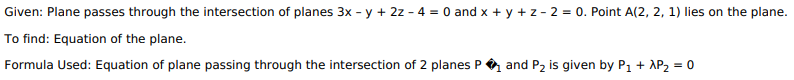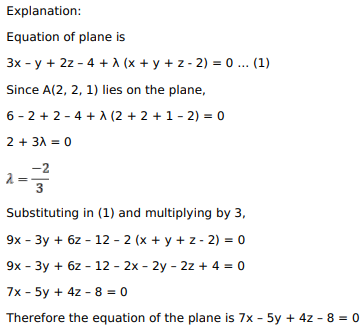# Mark against the correct answer in each of the following:

Question:

Mark against the correct answer in each of the following:

The equation of the plane passing through the intersection of the planes $3 x-y+2 z-4=0$ and $x+y+z-2=0$ and passing through the point $A(2,2,1)$ is given by

A. $7 x+5 y-4 z-8=0$

B. $7 x-5 y+4 z-8=0$

C. $5 x-7 y+4 z-8=0$

D. $5 x+7 y-4 z+8=0$

Solution: